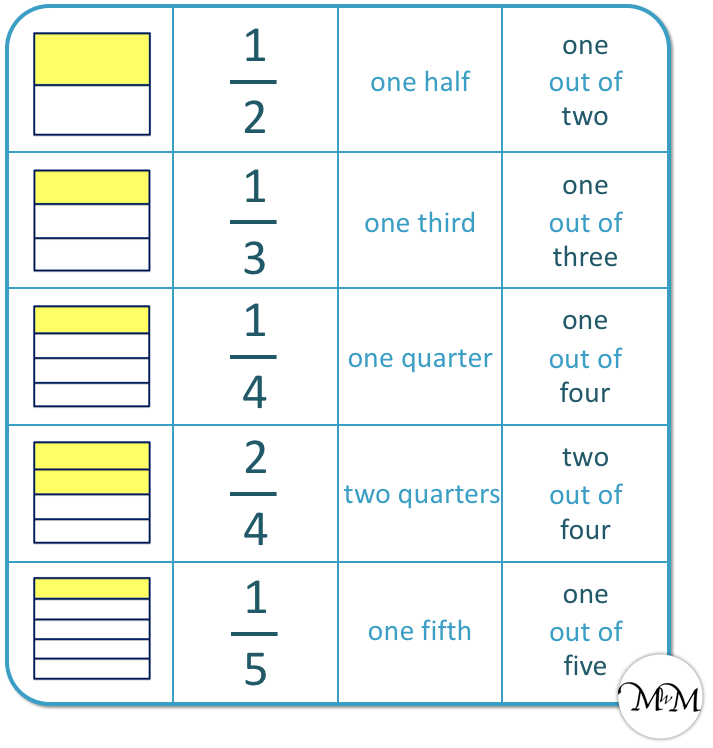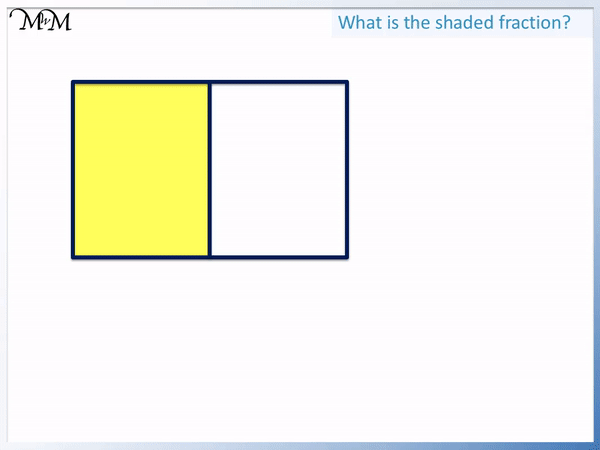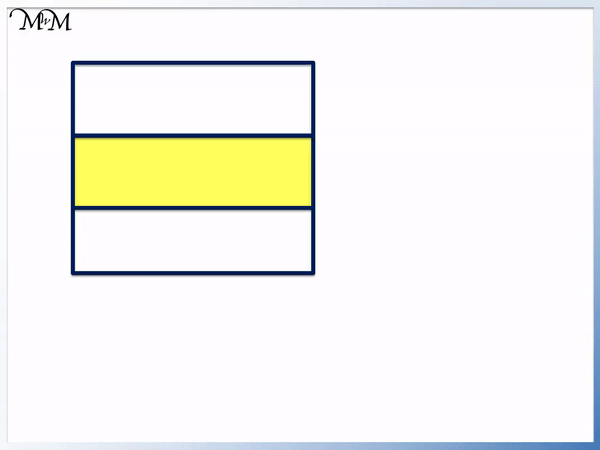# Identifying Simple Fractions

Identifying Simple Fractions• The number on the bottom of the fraction tells us how many parts a shape is divided into.
• The number on top of a fraction tells us how many of these parts we have shaded in.
•   1 / 2   is called one half.
•   1 / 3   is called one third.
•   1 / 4   is called one quarter.
•   2 / 4   is called two quarters.
•   1 / 5   is called one fifth.

The number of parts the shape is split into is written on the bottom of the fraction.

The number of shaded parts is written on the top of the fraction.• There is one shaded portion so we write a 1.
• We write a line under this line, which can be read as ‘out of’.
• The shape is divided into 3 parts in total, so we write a 3 below the line.
• We have   1 / 3  , which is called ‘one third’.
• We can say that we have 1 out of 3 equal parts.Supporting Lessons#### Identifying Simple Fractions of Shapes Interactive Question Generator

Identifying Simple Fractions: Interactive Questions

# How to Identify Simple Fractions of Shapes

## Naming Fractions

The number of parts that something has been divided into tells us the type of fraction we have. We then count how many of these parts we have.

If an amount is divided into 2 parts, these parts are called halves.

If an amount is divided into 3 parts, these are called thirds.

If an amount is divided into 4 parts, these are called quarters.

If an amount is divided into 5 parts, these are called fifths.

Here is a poster showing the names of some common fractions.## Identifying One Half

One half is an amount divided into two equal parts.

In the example below, the rectangle is divided into 2 equal parts, which are called halves.

To write one half as a fraction, we write a number above a dividing line with another number below the dividing line.We first count how many parts are shaded in. We have 1 part shaded in and so we can write a ‘1’.

We put our dividing line below this number.

Then we count how many parts the shape has been divided into in total. We have 2 parts.

We write a 2 below the dividing line.

We have   1 / 2   . The shape is divided into two equal parts, directly down the centre.

Here is another example of one half.

This triangle is divided into two equal parts down the centre.

We have one half shown shaded in.One half is written as   1 / 2   . The line in the fraction means to divide, so   1 / 2   means one whole divided by two.

## Identifying One Third

One third is an amount divided into 3 equal parts.

This rectangle is divided into 3 equal parts and so, it is divided into thirds.

We have one whole divided into 3, which can be written as   1 / 3   .We have one part shaded in and so we write a 1 above the dividing line. We have three parts in total so we write a 3 below the dividing line.

In this example we have one third of a circle.

We have one out of three equal parts shaded in.The fraction of one third can fit into the whole shape three times.

## Identifying One Quarter

One quarter is when something is divided into 4 equal parts.

In the example below, we have one out of four equal parts.

One divided by 4 can be written as   1 / 4   .We have one out of the four parts shown.

## Identifying Two Quarters

Two quarters is two out of four equal parts. Two quarters is the same as one half.

In the example below, two parts are shaded in. So we write a 2 above the dividing line.

There are 4 parts in total and so, we write a 4 below the dividing line.We have two of the four quarters.

Two out of four can be written as   2 / 4   .

We can see that if we remove one of the dividing lines in the shape, we have a rectangle split into 2 equal parts.

2 / 4   is the same size as   1 / 2   .Two quarters is the same as one half.

In the example below we have 2 parts shaded. We write a 2.

There are 4 parts in total and so, we write a 4 below the dividing line.

We have the fraction of   2 / 4   .We know that two quarters is the same as one half. We can see that we have half of the shape shaded in if we double it. When we add another two squares, the whole shape is filled in.

Two quarters make one half.

## Identifying One Fifth

One fifth is an amount is divided into five equal parts.

In the example below, the pentagon is divided into five equal parts.

We have 1 part shaded and so we write a 1 above the dividing line of the fraction.

There are five parts in total and so we write a 5 below the dividing line.One fifth is written as a fraction as   1 / 5   .

Here is another example of one fifth.

The circle below is divided into five equal parts.One fifth of the circle is shaded in.Now try our lesson on Writing Fractions of Geometric Shapes where we learn how to find further fractions of amounts.